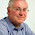## Monday, 26 May 2014

### A Public Domain C++11 1D/2D/3D Perlin Noise Generator

Recently I needed to generate some simple procedural textures for a game I'm working on - after a quick search I found that the top result was licensed under the GPL which is no good to me. After a while I did find an MIT licensed one, but as all these code snippets are just C++ ports of Ken Perlin's improved noise function written in Java, I figured I might as well write my own from scratch and license it under the public domain. So, here it is, in all its glory:

```#ifndef NOISE_H
#define NOISE_H

#include <random>
#include <array>

namespace noise {

class Perlin {
public:
Perlin(uint32_t seed=0);

double noise(double x) const { return noise(x, 0, 0); }
double noise(double x, double y) const { return noise(x, y, 0); }
double noise(double x, double y, double z) const;

private:
std::array<int, 512> p;
};

class PerlinOctave {
public:
PerlinOctave(int octaves, uint32_t seed=0);

double noise(double x) const { return noise(x, 0, 0); }
double noise(double x, double y) const { return noise(x, y, 0); }
double noise(double x, double y, double z) const;

private:
Perlin perlin_;
int octaves_;
};

}

#endif // NOISE_H
```
And the source file...
```#include <algorithm>

#include "noise.h"

namespace noise {

return t * t * t * (t * (t * 6 - 15) + 10);
}

double lerp(double t, double a, double b) {
return a + t * (b - a);
}

double grad(int hash, double x, double y, double z) {
int h = hash & 15;
double u = h < 8 ? x : y;
double v = h < 4 ? y : h == 12 || h == 14 ? x : z;
return ((h & 1) == 0 ? u : -u) + ((h & 2) == 0 ? v : -v);
}

Perlin::Perlin(uint32_t seed) {
if(!seed) {
seed = time(0);
}

auto mid_range = p.begin() + 256;

std::mt19937 engine(seed);

std::iota(p.begin(), mid_range, 0); //Generate sequential numbers in the lower half
std::shuffle(p.begin(), mid_range, engine); //Shuffle the lower half
std::copy(p.begin(), mid_range, mid_range); //Copy the lower half to the upper half
//p now has the numbers 0-255, shuffled, and duplicated
}

double Perlin::noise(double x, double y, double z) const {
//See here for algorithm: http://cs.nyu.edu/~perlin/noise/

const int32_t X = static_cast<int32_t>(std::floor(x)) & 255;
const int32_t Y = static_cast<int32_t>(std::floor(y)) & 255;
const int32_t Z = static_cast<int32_t>(std::floor(z)) & 255;

x -= std::floor(x);
y -= std::floor(y);
z -= std::floor(z);

const auto A = p[X] + Y;
const auto AA = p[A] + Z;
const auto AB = p[A + 1] + Z;
const auto B = p[X + 1] + Y;
const auto BA = p[B] + Z;
const auto BB = p[B + 1] + Z;

const auto PAA = p[AA];
const auto PBA = p[BA];
const auto PAB = p[AB];
const auto PBB = p[BB];
const auto PAA1 = p[AA + 1];
const auto PBA1 = p[BA + 1];
const auto PAB1 = p[AB + 1];
const auto PBB1 = p[BB + 1];

const auto a = lerp(v,
);

const auto b = lerp(v,
);

return lerp(w, a, b);
}

PerlinOctave::PerlinOctave(int octaves, uint32_t seed):
perlin_(seed),
octaves_(octaves) {

}

double PerlinOctave::noise(double x, double y, double z) const {
double result = 0.0;
double amp = 1.0;

int i = octaves_;
while(i--) {
result += perlin_.noise(x, y, z) * amp;
x *= 2.0;
y *= 2.0;
z *= 2.0;
amp *= 0.5;
}

return result;
}

}
```

It's pretty straightforward to use, just instantiate a Perlin, or PerlinOctave instance, and call noise(x, y, z); Simples.

To clarify, I'm unlicensing this code. Use it however you want for whatever you want.

1.Thanks for this! For those stuck with older compilers that don't support C++11, you can just add #include to the header, and replace the Perlin constructor with the following:

template
void my_random_shuffle(RandomIt first, RandomIt last)
{
typename std::iterator_traits::difference_type i, n;
n = last - first;
for (i = n-1; i > 0; --i) {
int tmp = first[i];
int dst = std::rand() % (i+1);
first[i] = first[dst];
first[dst] = tmp;
}
}

template
void my_iota (ForwardIterator first, ForwardIterator last, T val)
{
while (first!=last) {
*first = val;
++first;
++val;
}
}

Perlin::Perlin(uint32_t seed) {

auto mid_range = p.begin() + 256;

my_iota(p.begin(), mid_range, 0); //Generate sequential numbers in the lower half
my_random_shuffle(p.begin(), mid_range); //Shuffle the lower half
std::copy(p.begin(), mid_range, mid_range); //Copy the lower half to the upper half
//p now has the numbers 0-255, shuffled, and duplicated
}

2.Thanks for this. I needed something simple I could integrate into my project without adding library dependencies or GPL code and this was really helpful.

3.This is a great inspiring article.I am pretty much pleased with your good work.You put really very helpful information...
2k18codes.com

4.One of the most awaited this to happen in is to code you can always do that by opening the www.nba2kworld.net